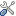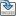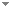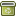# The complexity of oddan

Journal of Symbolic Logic 65 (1):1 - 18 (2000)

 Abstract For a fixed set A, the number of queries to A needed in order to decide a set S is a measure of S's complexity. We consider the complexity of certain sets defined in terms of A: $ODD^A_n = \{(x_1, \dots ,x_n): {\tt\#}^A_n(x_1, \dots, x_n) \text{is odd}\}$ and, for m ≥ 2, $\text{MOD}m^A_n = \{(x_1, \dots ,x_n):{\tt\#}^A_n(x_1, \dots ,x_n) \not\equiv 0 (\text{mod} m)\},$ where ${\tt\#}^A_n(x_1, \dots ,x_n) = A(x_1)+\cdots+A(x_n)$ . (We identify A(x) with χ A (x), where χ A is the characteristic function of A.) If A is a nonrecursive semirecursive set or if A is a jump, we give tight bounds on the number of queries needed in order to decide ODD A n and $\text{MOD}m^A_n: \bullet\text{ODD}^A_n$ can be decided with n parallel queries to A, but not with n - 1. $\bullet \text{ODD}^A_n$ can be decided with $\lceil log(n + 1)\rceil$ sequential queries to A but not with $\lceil log(n + 1)\rceil - 1. \bullet\text{MOD}m^A_n$ can be decided with $\lceil n/m\rceil + \lfloor n/m\rfloor$ parallel queries to A but not with $\lceil n/m\rceil + \lfloor n/m\rfloor - 1. \bullet\text{MOD}m^A_n$ can be decided with $\lceil log(\lceil n/m\rceil + \lfloor n/m\rfloor + 1)\rceil$ sequential queries to A but not with $\lceil log(\lceil n/m\rceil + \lfloor n/m\rfloor + 1)\rceil - 1$ . The lower bounds above hold for nonrecursive recursively enumerable sets A as well. (Interestingly, the lower bounds for recursively enumerable sets follow by a general result from the lower bounds for semirecursive sets.) In particular, every nonzero truth-table degree contains a set A such that ODD A n cannot be decided with n - 1 parallel queries to A. Since every truth-table degree also contains a set B such that ODD B n can be decided with one query to B, a set's query complexity depends more on its structure than on its degree. For a fixed set A, $Q(n,A) = \{S: S \text{can be decided with n sequential queries to} A\},\\Q_\parallel(n, A) = \{S: S \text{can be decided with n parallel queries to} A\}.$ We show that if A is semirecursive or recursively enumerable, but is not recursive, then these classes form non-collapsing hierarchies: $\bullet Q(0,A) \subset Q(1,A) \subset Q(2,A) \subset\cdots\\ \bullet Q_\parallel(0, A) \subset Q_\parallel(1, A) \subset Q_\parallel(2,A) \subset\cdots$ The same is true if A is a jump Keywords No keywords specified (fix it) Categories Logic and Philosophy of Logic (categorize this paper) DOI 10.2307/2586523 OptionsEdit this recordMark as duplicateExport citationFind it on ScholarRequest removal from indexRevision history

PhilArchive copy

Upload a copy of this paper     Check publisher's policy     Papers currently archived: 71,199

Setup an account with your affiliations in order to access resources via your University's proxy server
Configure custom proxy (use this if your affiliation does not provide a proxy)

## References found in this work BETA

No references found.

## Citations of this work BETA

No citations found.